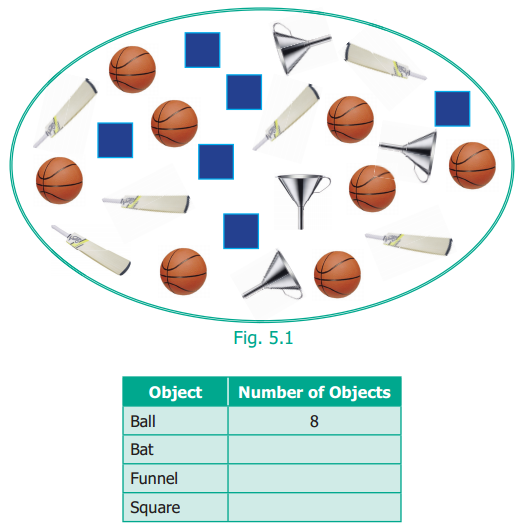## Tamilnadu Samacheer Kalvi 6th Maths Solutions Term 1 Chapter 5 Statistics Intext Questions

Recap (Textbook Page No. 108)

Question 1.
Count the objects in the following figure and complete the table that follows :From Fig 5.1 and the table, answer the following question.
(i) The total number of objects in the above picture is ______
(ii) The difference between the number of squares and the number of bats is ______
(iii) The ratio of the number of balls to the number of bats is _______
(iv) What are the objects equal in number?
(v) How many more balls are there than the number of bats?
Solution:
(i) 24
(ii) 0
(iii) $$\frac{8}{6}=\frac{4}{3}$$
(iv) Bat and Square
(v) 2 balls more